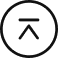回到顶部

# 【跨学科电学案例一】打开“电学之门” 简单

• 行空板 X1 链接
• Gravity: I2C数字功率计 X1 链接
• 面包板 X1 链接
• 光敏电阻及发光二极管 X1

电路在初中的科学、高中的物理和通用技术学科中都有所涉及，初中的串并联电路，高中物理的小灯泡伏安特性曲线、闭合电路欧姆定律等，高中通用技术的电子控制等都涉及到电压和电流，如何通过创客器材来给学生展示电流电压之间的关系，打开“电学之门”是我一直在思考的。

当然作为非信息教师使用，涉及的程序要尽量简单，器材尽量少，所以这扇门的打开不能太过复杂。在DF的创客商城找到了这个功率计，很好地解决了这个门的问题。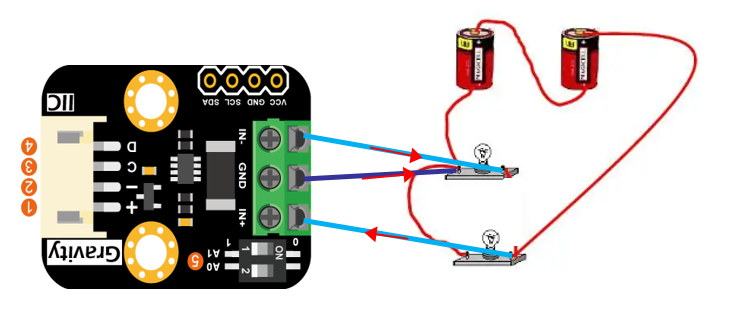将 in+和in- 是串联到负载电路中去，将GND则连接到负载的负极，这样就可以测负载的电流和他两端的电压。如上图就是测量上面一个灯泡的电流和电压。

接下来就是电流电压两者之间的关系研究，这里我想到了使用行空板，它能较好地显示数据等信息，且能够将数据图形化，更加直观明朗。

下面以通用技术中二极管为例进行研究：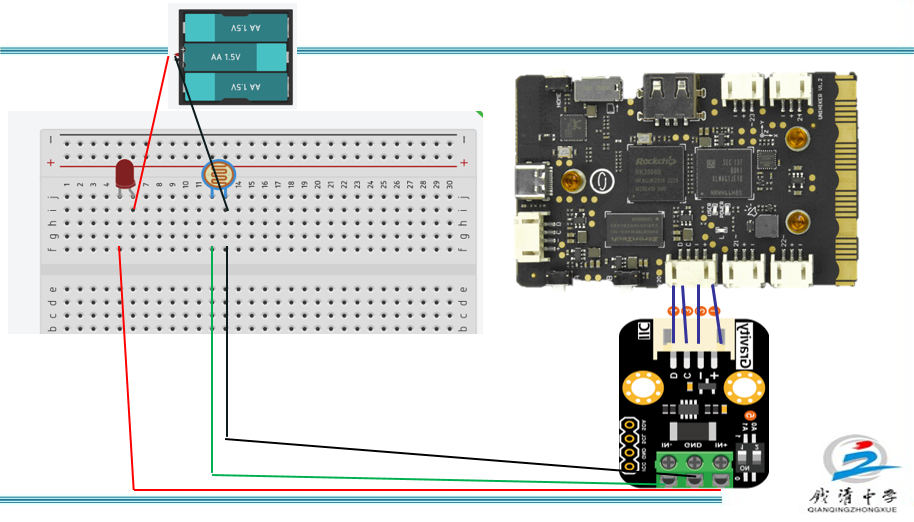2.1 引入matplotlib库

2.2  列表和变量

列表主要来收集数据，以便作图，变量B主要是进行切换界面。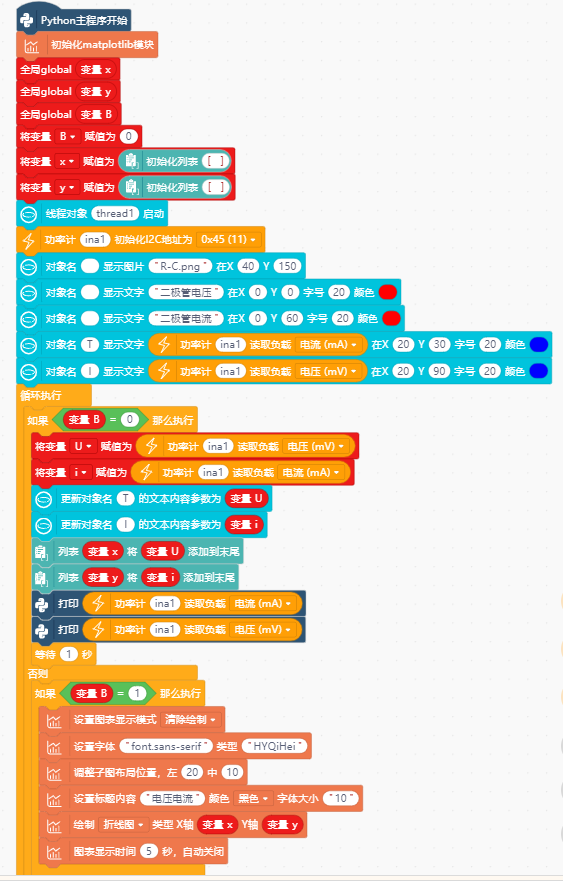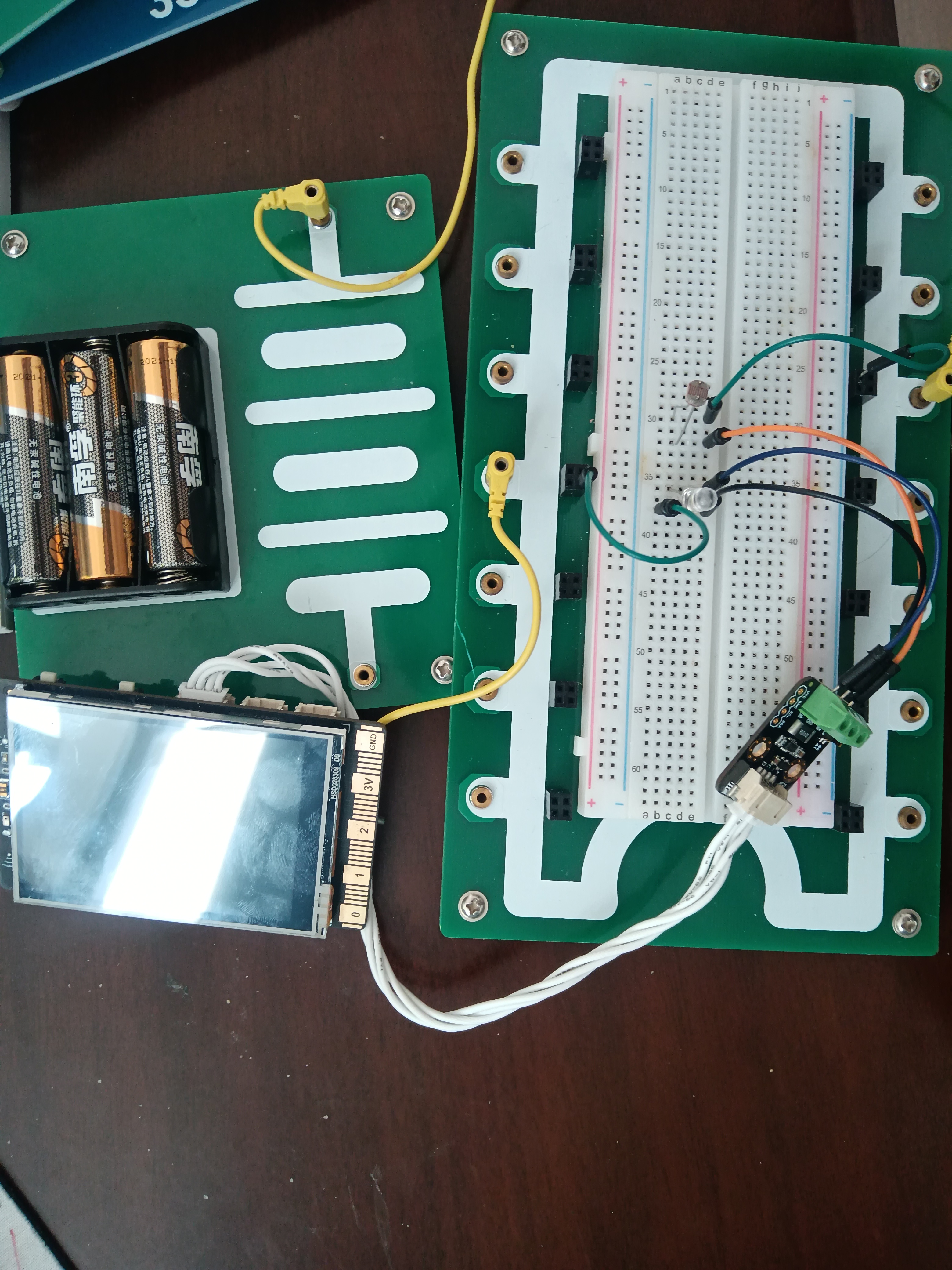实验的定性结果基本符合电子控制课本当中的U-I特性曲线，这也是做这个实验的目的。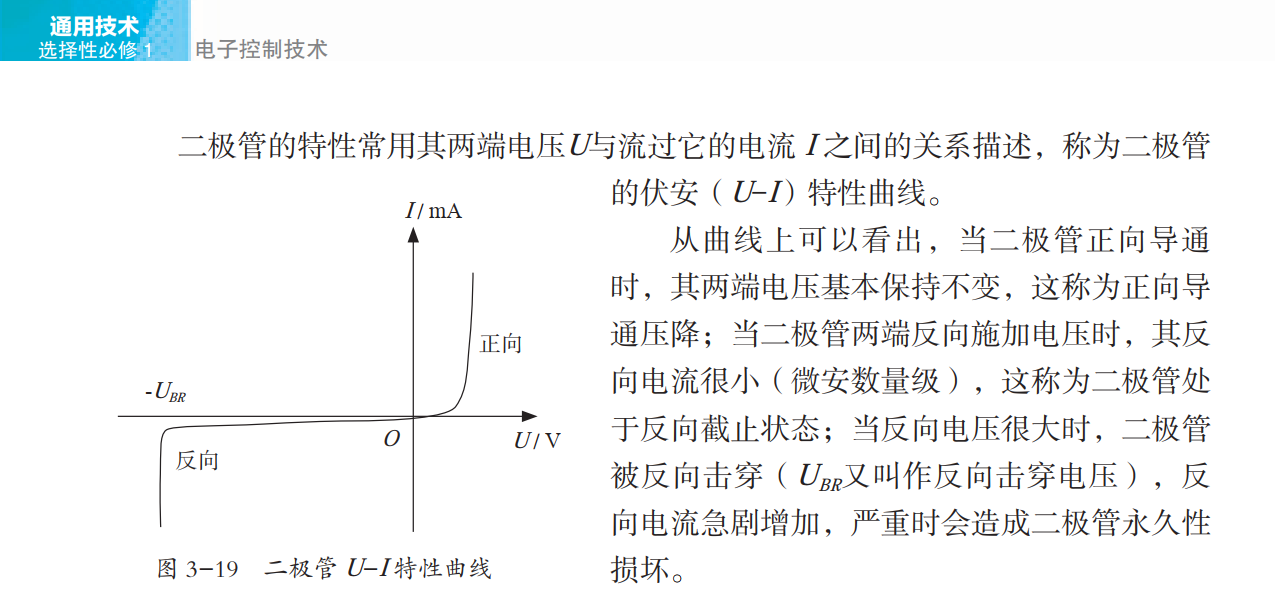当然这个实验中的面包板知识也是高中通用技术学科需要掌握的一个内容，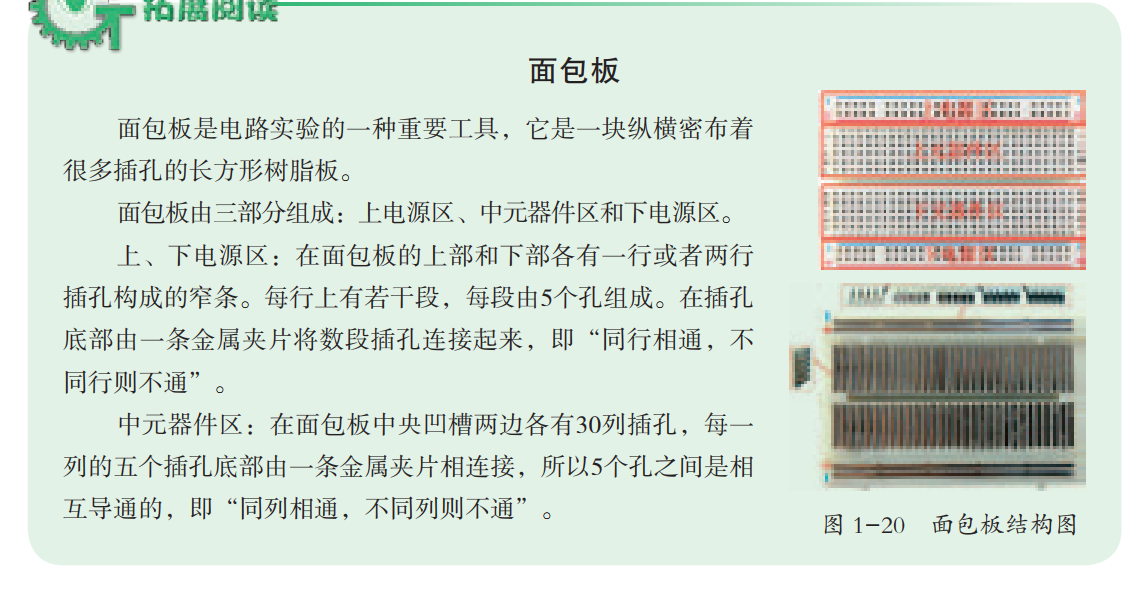通过这次尝试，我也发现了不少问题，有待后面的改进：

1.实验前没有进行校准

2.实验中光线变化不够连贯，导致数据不是非常连贯

3.程序中还存在一定错误，比如实验后要想重新测需要重新开启过程序，这个后面程序需要适当修改下，同时还不够美观

4.课本中的交流点实验没有尝试

但无论结果如何，都给我打开了通过行空板等设备来探究初高中学科中的知识的一扇门，相信后面通过自己的不断探索，能够将信息技术更好地应用到学科教学中去。

#  -*- coding: UTF-8 -*-

# MindPlus
# Python
from matplotlib import pyplot as plt
import matplotlib

from pinpong.libs.dfrobot_ina219 import INA219
from pinpong.extension.unihiker import *
from pinpong.board import Board,Pin
from pinpong.board import Board
from unihiker import GUI
import time

# 事件回调函数
global B
while True:
if (button_a.is_pressed()==True):
B = (B + 1)
if (B == 1):
T.remove()
I.remove()
P=u_gui.draw_text(text="数据处理中",x=20,y=20,font_size=20, color="#0000FF")
else:
if (B == 2):
P.remove()
B = 0

u_gui=GUI()
Board().begin()
global x
global y
global B
B = 0
x = []
y = []
ina1.begin()
u_gui.draw_image(image="R-C.png",x=40,y=150)
u_gui.draw_text(text="二极管电压",x=0,y=0,font_size=20, color="#FF0000")
u_gui.draw_text(text="二极管电流",x=0,y=60,font_size=20, color="#FF0000")
T=u_gui.draw_text(text=ina1.get_current_ma(),x=20,y=30,font_size=20, color="#0000FF")
I=u_gui.draw_text(text=ina1.get_bus_voltage_mv(),x=20,y=90,font_size=20, color="#0000FF")

while True:
if (B == 0):
U = ina1.get_bus_voltage_mv()
i = ina1.get_current_ma()
T.config(text=U)
I.config(text=i)
x.append(U)
y.append(i)
print(ina1.get_current_ma())
print(ina1.get_bus_voltage_mv())
time.sleep(1)
else:
if (B == 1):
plt.clf()
plt.rcParams["font.sans-serif"] = ["HYQiHei"]